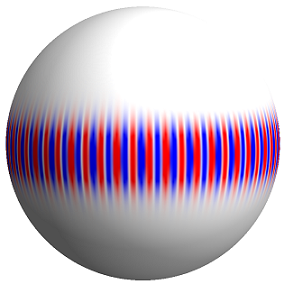# Research Theme: Short-Wavelength Quasinormal Modes

This post describes two papers and a comment about quasinormal modes of Kerr black holes in the short-wavelength (geometric-optics) limit.

## Papers Highlighted

• A. Zimmerman, H. Yang, F. Zhang, D. A. Nichols, E. Berti, and Y. Chen. “Reply to ‘On the branching of quasinormal resonances of near-extremal Kerr black holes’ by Shahar Hod.” arXiv:1510.08159.

• H. Yang, F. Zhang, A. Zimmerman, D. A. Nichols, E. Berti, and Y. Chen. “Branching of quasinormal modes for nearly extremal Kerr black holes.” Phys. Rev. D. 87, 041502(R) (2013), arXiv:1212.3271.

• H. Yang, D. A. Nichols, F. Zhang, A. Zimmerman, Z. Zhang, and Y. Chen. “Quasinormal-mode spectrum of Kerr black holes and its geometric interpretation.” Phys. Rev. D 86, 104006 (2012), arXiv:1207.4253.

## Summary of the PapersPerturbed black holes oscillate at characteristic frequencies and with amplitudes that decay at specific rates in what are called quasinormal modes. For non-rotating and slowly rotating modes, it was noted that the oscillation frequency of short-wavelength modes was related to the orbital and precessional frequencies of null geodesics that remain at fixed radius (on the photon sphere). The spherical photon orbits are unstable, and they head out or into the black hole on a time scale given by the Lyapunov exponent, which is equivalent to the rate of decay for short-wavelength quasinormal modes. The reason for this apparent coincidence is that quasinormal modes are massless disturbances propagating on the black hole’s background, and in the short-wavelength (geometric-optics) limit, the mode follows the null rays (geodesics) of the spacetime (as would any other massless field).

We showed that the correspondence holds for Kerr black holes of any physical spin by explicitly comparing the equations of geometric optics and those of black-hole perturbations in the short-wavelength limit and relating the two sets of parameters in the two complementary descriptions. Our analysis also provided a method to calculate quasinormal-mode frequencies approximately with good accuracy. When applied to black holes with nearly maximal spin angular momentum, we were able to observe a splitting in the spectrum of the modes as the damping rate of the modes went to zero. This is likely connected to the fact that black holes with exactly maximal spins are marginally stable.

Categories:

Updated: